## Binary Search Tree-

Before you go through this article, make sure that you have gone through the previous article on Binary Search Trees.

Binary search tree (BST) is a special kind of binary tree where each node contains-

• Only larger values in its right subtree.
• Only smaller values in its left subtree.

## BST Traversal-

• A binary search tree is traversed in exactly the same way a binary tree is traversed.
• In other words, BST traversal is same as binary tree traversal.

## Example-

Consider the following binary search tree-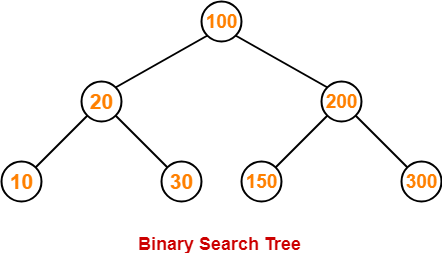Now, let us write the traversal sequences for this binary search tree-

### Preorder Traversal-

100 , 20 , 10 , 30 , 200 , 150 , 300

### Inorder Traversal-

10 , 20 , 30 , 100 , 150 , 200 , 300

### Postorder Traversal-

10 , 30 , 20 , 150 , 300 , 200 , 100

## Note-01:

• Inorder traversal of a binary search tree always yields all the nodes in increasing order.

## Note-02:

Unlike Binary Trees,

• A binary search tree can be constructed using only preorder or only postorder traversal result.
• This is because inorder traversal can be obtained by sorting the given result in increasing order.

To gain better understanding about Binary Search Tree Traversal,

Watch this Video Lecture

## Problem-01:

Suppose the numbers 7 , 5 , 1 , 8 , 3 , 6 , 0 , 9 , 4 , 2 are inserted in that order into an initially empty binary search tree. The binary search tree uses the usual ordering on natural numbers.

What is the inorder traversal sequence of the resultant tree?

1. 7 , 5 , 1 , 0 , 3 , 2 , 4 , 6 , 8 , 9
2. 0 , 2 , 4 , 3 , 1 , 6 , 5 , 9 , 8 , 7
3. 0 , 1 , 2 , 3 , 4 , 5 , 6 , 7 , 8 , 9
4. 9 , 8 , 6 , 4 , 2 , 3 , 0 , 1 , 5 , 7

## Solution-

This given problem may be solved in the following two ways-

### Method-01:

• We construct a binary search tree for the given elements.
• We write the inorder traversal sequence from the binary search tree so obtained.

Following these steps, we have-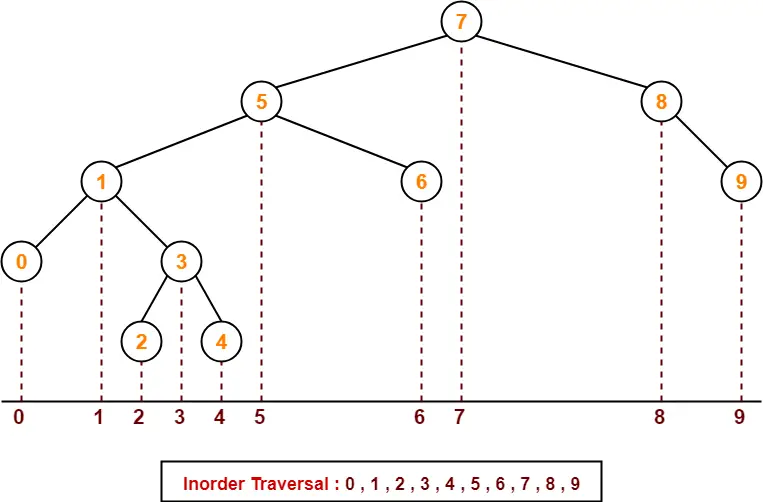Thus, Option (C) is correct.

### Method-02:

We know, inorder traversal of a binary search tree always yields all the nodes in increasing order.

Using this result,

• We arrange all the given elements in increasing order.
• Then, we report the sequence so obtained as inorder traversal sequence.

 Inorder Traversal : 0 , 1 , 2 , 3 , 4 , 5 , 6 , 7 , 8 , 9

Thus, Option (C) is correct.

## Problem-02:

The preorder traversal sequence of a binary search tree is-

30 , 20 , 10 , 15 , 25 , 23 , 39 , 35 , 42

What one of the following is the postorder traversal sequence of the same tree?

1. 10 , 20 , 15 , 23 , 25 , 35 , 42 , 39 , 30
2. 15 , 10 , 25 , 23 , 20 , 42 , 35 , 39 , 30
3. 15 , 20 , 10 , 23 , 25 , 42 , 35 , 39 , 30
4. 15 , 10 , 23 , 25 , 20 , 35 , 42 , 39 , 30

## Solution-

In this question,

• We are provided with the preorder traversal sequence.
• We write the inorder traversal sequence by arranging all the numbers in ascending order.

Then-

• Postorder Traversal : 30 , 20 , 10 , 15 , 25 , 23 , 39 , 35 , 42
• Inorder Traversal : 10 , 15 , 20 , 23 , 25 , 30 , 35 , 39 , 42

Now,

• We draw a binary search tree using these traversal results.
• The binary search tree so obtained is as shown-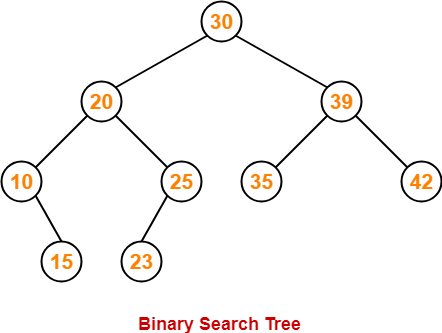Now, we write the postorder traversal sequence-

 Postorder Traversal : 15 , 10 , 23 , 25, 20, 35, 42, 39, 30

Thus, Option (D) is correct.

To watch video solutions and practice more problems,

Watch this Video LectureNext Article- Binary Search Tree Operations

Get more notes and other study material of Data Structures.

Watch video lectures by visiting our YouTube channel LearnVidFun.

## Binary Tree-

Before you go through this article, make sure that you gone through the previous article on Binary Trees.

We have discussed-

• Binary tree is a special tree data structure.
• In a binary tree, each node can have at most 2 children.

## Tree Traversal-

 Tree Traversal refers to the process of visiting each node in a tree data structure exactly once.

Various tree traversal techniques are-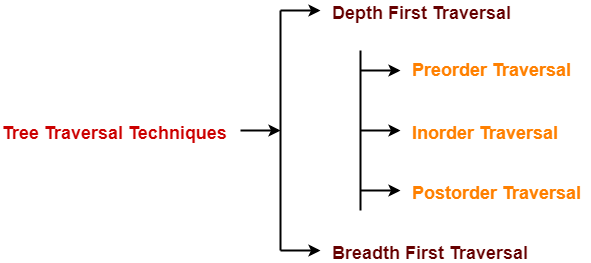## Depth First Traversal-

Following three traversal techniques fall under Depth First Traversal-

1. Preorder Traversal
2. Inorder Traversal
3. Postorder Traversal

## Algorithm-

1. Visit the root
2. Traverse the left sub tree i.e. call Preorder (left sub tree)
3. Traverse the right sub tree i.e. call Preorder (right sub tree)

Root  Left  Right

## Example-

Consider the following example-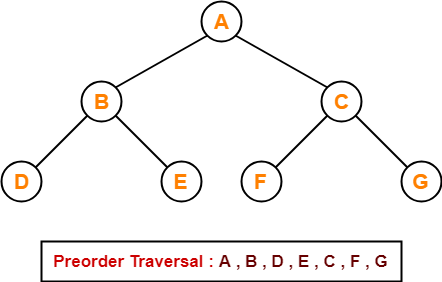## Preorder Traversal Shortcut

Traverse the entire tree starting from the root node keeping yourself to the left.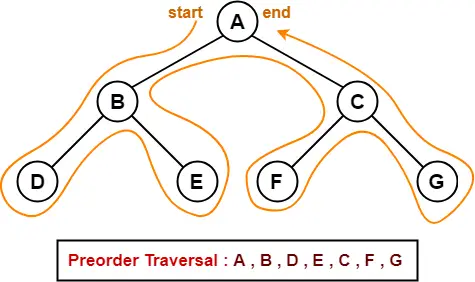## Applications-

• Preorder traversal is used to get prefix expression of an expression tree.
• Preorder traversal is used to create a copy of the tree.

## Algorithm-

1. Traverse the left sub tree i.e. call Inorder (left sub tree)
2. Visit the root
3. Traverse the right sub tree i.e. call Inorder (right sub tree)

Left  Root  Right

## Example-

Consider the following example-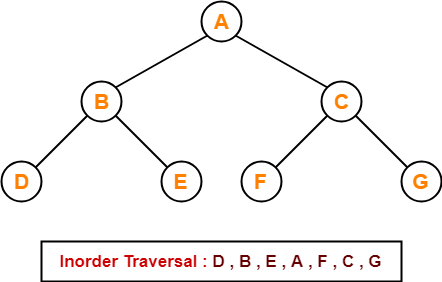## Inorder Traversal Shortcut

Keep a plane mirror horizontally at the bottom of the tree and take the projection of all the nodes.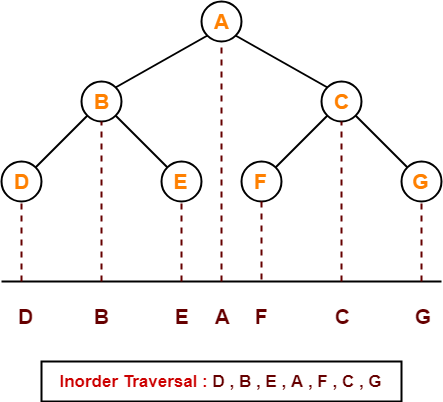## Application-

• Inorder traversal is used to get infix expression of an expression tree.

## Algorithm-

1. Traverse the left sub tree i.e. call Postorder (left sub tree)
2. Traverse the right sub tree i.e. call Postorder (right sub tree)
3. Visit the root

Left  Right  Root

## Example-

Consider the following example-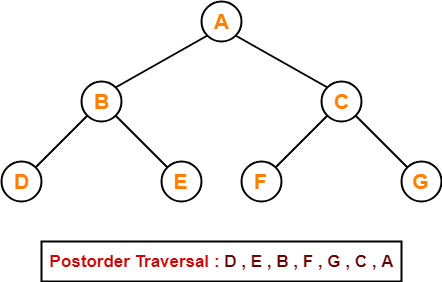## Postorder Traversal Shortcut

Pluck all the leftmost leaf nodes one by one.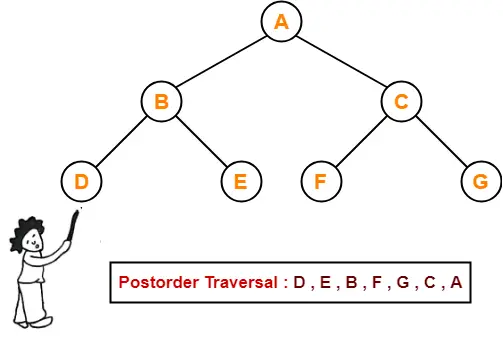## Applications-

• Postorder traversal is used to get postfix expression of an expression tree.
• Postorder traversal is used to delete the tree.
• This is because it deletes the children first and then it deletes the parent.

• Breadth First Traversal of a tree prints all the nodes of a tree level by level.
• Breadth First Traversal is also called as Level Order Traversal.

## Example-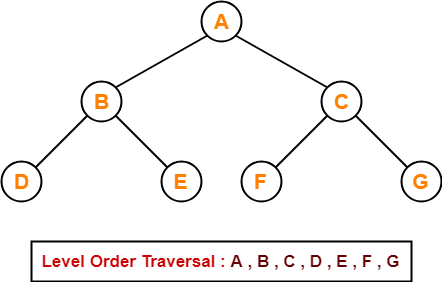## Application-

• Level order traversal is used to print the data in the same order as stored in the array representation of a complete binary tree.

To gain better understanding about Tree Traversal,

Watch this Video Lecture

## Problem-01:

If the binary tree in figure is traversed in inorder, then the order in which the nodes will be visited is ____?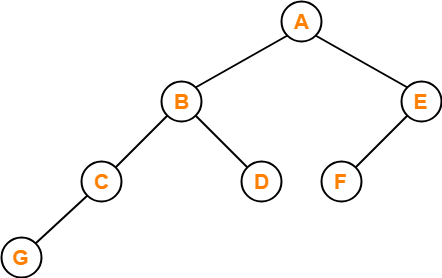## Solution-

The inorder traversal will be performed as-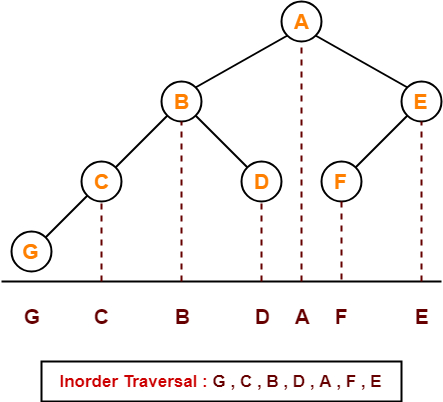## Problem-02:

Which of the following sequences denotes the postorder traversal sequence of the tree shown in figure?1. FEGCBDBA
2. GCBDAFE
3. GCDBFEA
4. FDEGCBA

## Solution-

Perform the postorder traversal by plucking all the leftmost leaf nodes one by one.

Then,

Postorder Traversal :  G , C , D , B , F , E , A

Thus, Option (C) is correct.

## Problem-03:

Let LASTPOST, LASTIN, LASTPRE denote the last vertex visited in a postorder, inorder and preorder traversal respectively of a complete binary tree. Which of the following is always true?

1. LASTIN = LASTPOST
2. LASTIN = LASTPRE
3. LASTPRE = LASTPOST
4. None of these

## Solution-

Consider the following complete binary tree-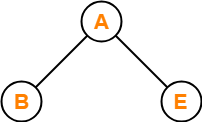Preorder Traversal  : B , A , E

Inorder Traversal     : B , A , E

Postorder Traversal : B , E , A

Clearly, LASTIN = LASTPRE.

Thus, Option (B) is correct.

## Problem-04:

Which of the following binary trees has its inorder and preorder traversals as BCAD and ABCD respectively-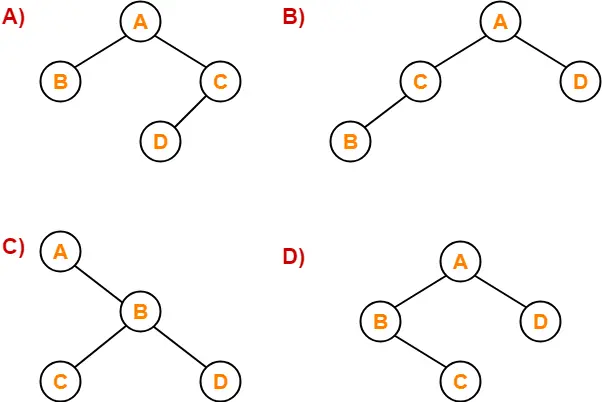## Solution-

Option (D) is correct.

To watch video solutions and practice more problems,

Watch this Video LectureNext Article- Binary Search Trees

Get more notes and other study material of Data Structures.

Watch video lectures by visiting our YouTube channel LearnVidFun.

[mtouchquiz 1]